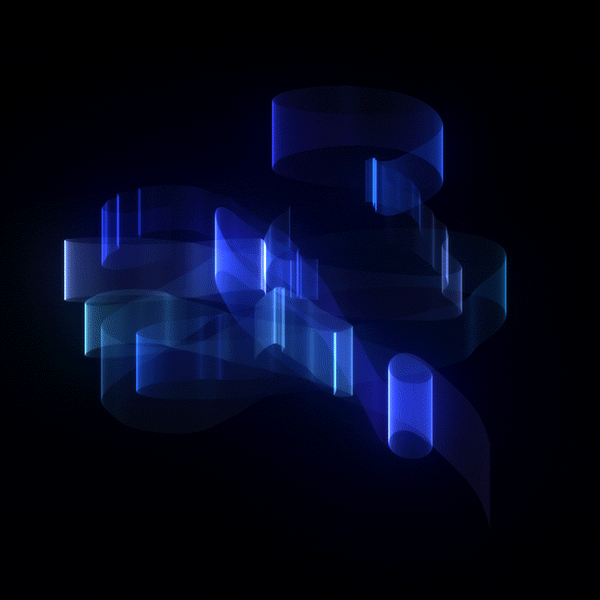###BettyES twitter logo github logo Feb 14 '18・4 min read

I have recently written a very basic beginner's guide to a simple shiny app. To be found here

The intention was that I had a set of results (differing by the value of one input variable) that I wanted to compare. After some tiresome rerunning the model and plotting results for comparisons I decided to make the whole process a bit quicker.

Thanks to shiny adjustment of the input values created results at an instant, made the whole interactive and more fun to present.

Sounds good? Ok, lets do it:

Some very basic introduction to build your shiny app.

## Get started...

To start off install.packages("shiny"). The two main components of your first shiny app are the ui (user interface) and server (holds the server-side logic - the server function). Basically you take care of all your designs in the ui and add all your functionality in the server (user input ==> outputs). Now two options are available:
1-store files separately in one folder as ui.R and server.R
2-store them in one file (in this case make sure to add shinyApp(ui = ui,
server = server) in the end).

``````library(shiny)
ui <- fluidPage()
server <- function(input, output) {}
shinyApp(ui = ui, server = server)
``````

I think shiny appears to be incredibly easy in creating webapps with lots of functionality and great looks, from what I have seen so far elsewhere (python, java). Though I have not tried out a lot in these languages and am far from confident in building python or java webapps.

To create a nice user interface shiny offers some easy to use (HTML derived) commands:
strong() for bold text,
em() for italicized text,
br() to add a line break,
code() to display,
hr() for a horizontal rule.

Have a look here for more options.

## Layout

There are several layout options:

### 1- Default layout...

...is to have a sidebar for inputs and a major panel for outputs (sidebarpanel(input), mainbarpanel(output) within the ui)

``````library(shiny)
library(ggplot2)
ui <- fluidPage(
h1("Some iris data visualised"),br(),

sidebarLayout(position="left",

sidebarPanel(
sliderInput("bin", "Binwidth:",min = 0.1, max = 1, value = 1)
),

mainPanel(plotOutput("IrisPlot"))
)
)

server <- function(input, output) {
output\$IrisPlot <- renderPlot({
ggplot(iris)+geom_histogram(aes(Sepal.Length, fill=Species),
binwidth=input\$bin, colour="white")+facet_wrap(~Species)
})
}

shinyApp(ui = ui, server = server)
``````

### 2- Grid layout...

...uses fluidrow(), columns() and wellpanel(). The page is separated into 12 columns, so make sure to add up to these. The wellpanel() creates a panel with grey background. To create the same image as above use:

``````library(shiny)
library(ggplot2)
ui <- fluidPage(
h1("Some iris data visualised"),br(),

fluidRow(
column(4,
wellPanel(
sliderInput("bin", "Binwidth:",min = 0.1, max = 1, value = 1)
)
),

column(8,
plotOutput("IrisPlot")
)
)
)

server <- function(input, output) {
output\$IrisPlot <- renderPlot({
ggplot(iris)+geom_histogram(aes(Sepal.Length, fill=Species),
binwidth=input\$bin, colour="white")+facet_wrap(~Species)
})
}
``````

### 3- Layered layout...

...with tabsetPanel() and navlistPanel()

``````library(shiny)
library(ggplot2)
ui <- fluidPage(
h1("Some iris data visualised"),br(),
fluidRow(
column(4,
wellPanel(
sliderInput("bin", "Binwidth:",
min = 0.1, max = 1, value = 1)
)),

column(8,
tabsetPanel(
tabPanel("Plot",plotOutput("IrisPlot")),
tabPanel("Summary",verbatimTextOutput("IrisSummary")),
tabPanel("Table",tableOutput("IrisTable"))
))
)
)
server <- function(input, output) {
output\$IrisPlot <- renderPlot({
ggplot(iris)+geom_histogram(aes(Sepal.Length,         fill=Species),binwidth=input\$bin, colour="white")+facet_wrap(~Species)
})
output\$IrisSummary <- renderPrint({summary(iris)})
output\$IrisTable <-renderTable(iris)
}
shinyApp(ui = ui, server = server)
``````

If you have more than a few tabs the navlistPanel may come in handy, adding sidebars instead of tabs to your shiny app. the code is the same as above, just replace tabsetPanel with navlistPanel.

### 4- Layout with several pages...

...navbarPage() will do the trick. "Just" replace your fluidPage() with navbarPage(), well..., I admit that is not all. You will have to restructure a bit, as shown below:

``````library(shiny)
library(ggplot2)
ui <-navbarPage("Iris data: ",
tabPanel("Plot",
sidebarLayout(position="left",
sidebarPanel(
sliderInput("bin", "Binwidth:",min = 0.1, max = 1, value = 1)
),
mainPanel(
plotOutput("IrisPlot")
))
),
tabPanel("Summary",
verbatimTextOutput("IrisSummary")),
tabPanel("Table",
tableOutput("IrisTable"))
)

server <- function(input, output) {
output\$IrisPlot <- renderPlot({
ggplot(iris)+geom_histogram(aes(Sepal.Length, fill=Species),
binwidth=input\$bin, colour="white")+facet_wrap(~Species)
})
output\$IrisSummary <- renderPrint({summary(iris)})
output\$IrisTable <-renderTable(iris)
}

shinyApp(ui = ui, server = server)
``````

### Shiny done

Feel free to add more widgets (please have a look here for the vast amount of choices) and off you go with your first basic shiny Appcode below...

``````library(shiny)
library(ggplot2)
ui <- fluidPage(
h1("Some iris data visualised"),br(),
fluidRow(
column(4,
wellPanel(
sliderInput("bin", "Binwidth:",
min = 0.1, max = 1, value = 1)
)),
column(8,
tabsetPanel(
tabPanel("Plot",plotOutput("IrisPlot")),
tabPanel("Summary",verbatimTextOutput("IrisSummary")),
tabPanel("Table",tableOutput("IrisTable"))
))
),
fluidRow(
column(4,
checkboxGroupInput("Species", label = "Select Species",
choices = list("Iris setosa" = "setosa",
"iris versicolor" = "versicolor",
"iris verginica" = "virginica"),
selected = 1)),
column(8,plotOutput("IrisPlot2"))
)
)

server <- function(input, output) {
output\$IrisPlot <- renderPlot({
ggplot(iris)+geom_histogram(aes(Sepal.Length,     fill=Species),binwidth=input\$bin, colour="white")+facet_wrap(~Species)
})
output\$IrisSummary <- renderPrint({summary(iris)})
output\$IrisTable <-renderTable(iris)

output\$IrisPlot2 <- renderPlot({
ggplot(iris[iris\$Species==input\$Species,])+geom_point(aes(Sepal.Length,     Sepal.Width,color=Species))
})
}

shinyApp(ui = ui, server = server)
``````
DISCUSSdev.to now has dark mode.

Select night theme in the "misc" section of your settings ❤️

Classic DEV Post from Oct 29 '17

## Daily git usage